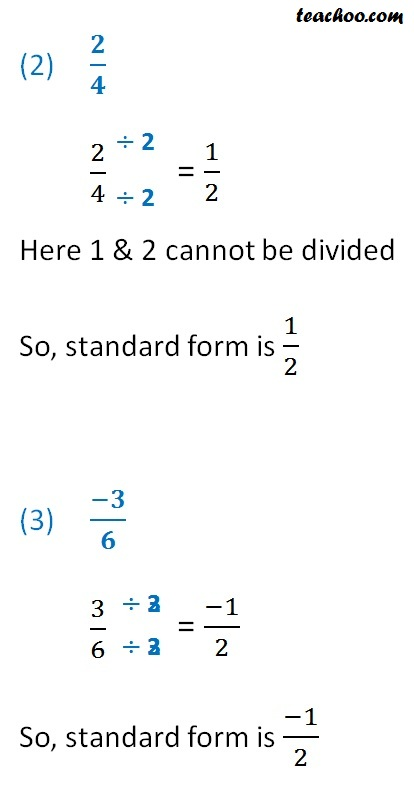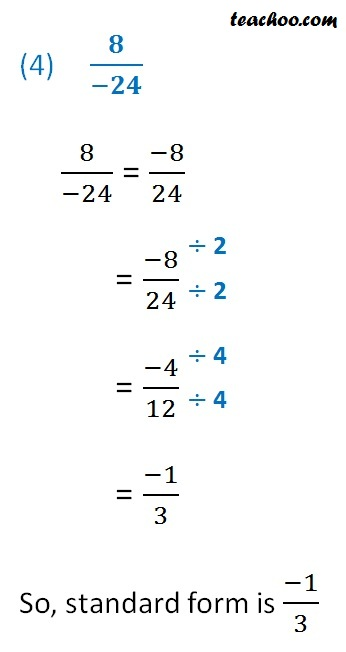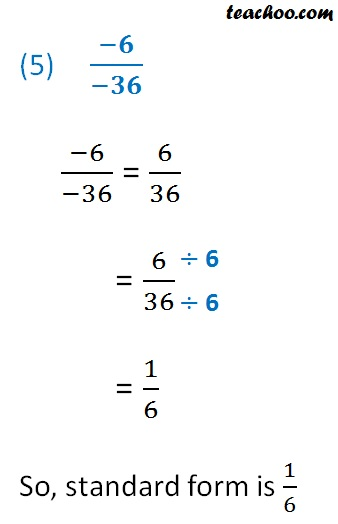Standard form of rational numbers

Chapter 8 Class 7 Rational Numbers
Concept wise

In standard form of rational number

• Denominator doesn’t have negative sign
• Fraction are simplified so that numerator & denominator cannot be smaller.

Let’s take some examples

#### (1)   2/(-3)

2/(-3) = (-2)/3

Here 2 & 3 cannot be divided

So, standard form is (-2)/3Learn in your speed, with individual attention - Teachoo Maths 1-on-1 Class

### Transcript

2) 𝟐/𝟒 2/4 Here 1 & 2 cannot be divided So, standard form is 1/2 (3) (−𝟑)/𝟔 3/6 So, standard form is (−1)/2 (4) 𝟖/(−𝟐𝟒) 8/(−24) = (−8)/24 = (−8)/24 = (−4)/12 = (−1)/3 So, standard form is (−1)/3 (5) (−𝟔)/(−𝟑𝟔) (−6)/(−36) = 6/36 = 6/36 = 1/6 So, standard form is 1/6